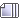###Author Topic: Impossible Math  (Read 3141 times)

#### Inv3rted

I see your logic, but it's an issue of semantics as to whether the problem is broken or not. When you divide by the same number on both sides of the equation, even if it is undefined, the equality remains true.

#### Riot

Exponents.
x2

aah, great I know what you ment but i never learned that sign

#### Rughugger

I find this topic hilarious, you're asking kids who haven't even gotten to basic algebra about exponents and things like that. Oh you slay me, mighty troll.

#### Jsk2003

x = y
x^2 = xy
x^2-y^2 = xy-y^2
(x+y)(x-y) = y(x-y)
x+y = y

2y = y
2 = 1
1 = 0
But, the only number that x can equal is clearly 0.
Damn cancelling of variables.  It's clearly broken.
You divided by (x-y) and x=y, thus you divided by zero.

So you're wrong.

#### Muffinmix

Guys. Zeros don't vanish.

(x+y)(x-y) = y(x-y)
assuming y=x
(x+x)(x-x) = x(x-x)
(x+x)(0) = x(0)
0 = 0

What's the problem?

Also, if you divide both sides by (x-y)

(x+y) = y

This still works if x = y = 0, or if unlike in this problem, x =/= y and x = 0

« Last Edit: July 29, 2009, 11:45:38 AM by Muffinmix »

#### FlyGuy45

I've seen this problem before, or something like it, its just a hidden / by zero proof of some sort.

#### AGlass0fMilk

They are imaginary numbers because

if x=y, then x+y cannot possibly = y

They are imaginary numbers, thus they don't really exist.

#### Inv3rted

They are imaginary numbers because

if x=y, then x+y cannot possibly = y

They are imaginary numbers, thus they don't really exist.

LOL.

Try making them both 0, you loving idiot.

#### AGlass0fMilk

Oh.

If they are both 0, then it's all just 0. I don't see why it's so hard then.

Dividing by 0 would just equal that number since there is nothing to divide by, or would it equal 0? Since they are all 0 it would equal zero anyways, or would it?
« Last Edit: July 29, 2009, 11:53:26 AM by AGlass0fMilk »

#### FlyGuy45

Oh.

If they are both 0, then it's all just 0. I don't see why it's so hard then.

Dividing by 0 would just equal that number since there is nothing to divide by, or would it equal 0? Since they are all 0 it would equal zero anyways, or would it?

LOL.

#### AGlass0fMilk

I found the answer, it equals:01000001010000010100000101000 001010000010100000101000001 AHHHHHHHH

#### FlyGuy45

I found the answer, it equals:The quickest way out of a hole is to stop digging.

The quickest way out of a hole is to stop digging.
Wait a minute.  If you've dug a 20 feet deep hole, even if you stop digging, you still won't be getting out of it (unless you can rocket jump.)

#### AGlass0fMilk

Can you throw me a rope or something?

#### Muffinmix

I think the part that confuses people is this line which comes after cases in which any variable you switch with y or x (keeping in mind y = x) should also accept any variable. But it's a completely different equation.

(x+y)(x-y) = y(x-y)

The math is impossible because the equation is already limited to only one possible outcome, which is x = y = 0, unlike the previous equations where x = y = anything-under-the-sun, and so you need to start paying attention to what your operations do simultaneous to other operations. Here's the kicker, which can be looked at from a different angle other then "Omg divide by zero"

When you perform the elimination to get from the first step to the second step here,

2y = y
(2y / y = y / y)
2 = 1

You are simultaneously assuming y can be substituted as 1 through a similar operation with the exact same result

2y = y
2(1) = (1)
2 = 1

which is impossible as you can see from the inequality and further up the equation chain. It is possible if you eliminate y by substituting it as zero, but other then that an elimination operation like that division breaks the rules by simultaneously stating x = y = 1.